##### Trigonometry Workbook For DummiesMultiple-angle trigonometry expressions are those where the angle measure is some multiple of a variable — for example, 2x or 3y. You can take these expressions apart and solve for all the additional solutions that are possible. Because the trig functions are periodic (meaning they repeat their patterns infinitely), the number of possibilities for solutions increases tremendously. The larger the multiplier, the more solutions.

When solving a trig equation of the form ax = f1(k) where you want the solution to be all the angles within one complete rotation, write out all the solutions within the number of complete rotations that k represents. Then divide each angle measure by a.

Problems that lend themselves to this technique are those such as 2sin25x = 1 and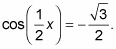In the first example, you solve 2sin25x = 1 for all the angles between 0 and 2π.

1. Divide each side by 2; then take the square root of each side.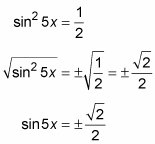2. Solve for 5x, which represents the angles that satisfy the equation within one rotation.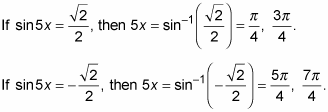3. Extend the solutions to five rotations by adding 2π to each of the original angles four times.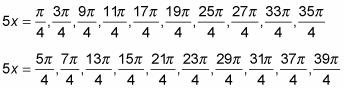4. Divide all the terms by 5 and simplify.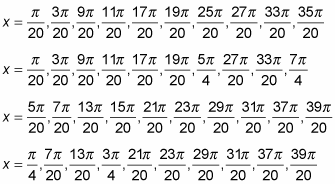Notice that all 16 solutions are angles with measures less than 2π.

This next example has a proper-fraction multiplier rather than a multiplier greater than 1.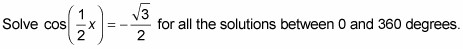1. Rewrite the equation as an inverse trig equation.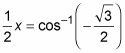2. Determine which angles satisfy the inverse equation within one full rotation.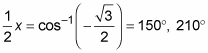3. Multiply all the terms by 2.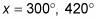4. Throw out the second angle, because its measure is greater than 360 degrees.

The only solution is 300 degrees. When you replace the x in the original equation with this angle measure, you get a true statement.